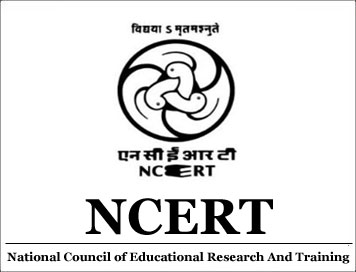# Class 5

... More

NCERT Solutions for Class 5 Maths (Math Magic) Chapter 1 The fish Tale

Get here NCERT Solution for Class 5 Maths Chapter 1 for Free. NCERT Solutions for Maths Class 5 provided here are best answers by AglaSem School experts, who have many year of experience in this field. Class 5 NCERT Textbook Solutions cover all the exercises of each chapter. NCERT Solutions for Class 5 Maths Math Magic Book are given below.
Book Name: Math Magic
Subject: Maths
Chapter Number: 1
Chapter Name: The fish Tale
You can also bookmark this page by clicking + Save to Library icon and refer to these NCERT Solutions anytime from your account.

NCERT Solutions for Class 5 Maths (Math Magic) Chapter 3 How Many Squares?

Get here NCERT Solution for Class 5 Maths Chapter 3 for Free. NCERT Solutions for Maths Class 5 provided here are best answers by AglaSem School experts, who have many year of experience in this field. Class 5 NCERT Textbook Solutions cover all the exercises of each chapter. NCERT Solutions for Class 5 Maths Math Magic Book are given below.
Book Name: Math Magic
Subject: Maths
Chapter Number: 3
Chapter Name: How Many Squares?
You can also bookmark this page by clicking + Save to Library icon and refer to these NCERT Solutions anytime from your account.

NCERT Solutions for Class 5 Maths (Math Magic) Chapter 2 Shapes and Angles

Get here NCERT Solution for Class 5 Maths Chapter 2 for Free. NCERT Solutions for Maths Class 5 provided here are best answers by AglaSem School experts, who have many year of experience in this field. Class 5 NCERT Textbook Solutions cover all the exercises of each chapter. NCERT Solutions for Class 5 Maths Math Magic Book are given below.
Book Name: Math Magic
Subject: Maths
Chapter Number: 2
Chapter Name: Shapes and Angles
You can also bookmark this page by clicking + Save to Library icon and refer to these NCERT Solutions anytime from your account.

NCERT Solutions for Class 5 Maths (Math Magic) Chapter 5 Does it Look the Same?

Get here NCERT Solution for Class 5 Maths Chapter 5 for Free. NCERT Solutions for Maths Class 5 provided here are best answers by AglaSem School experts, who have many year of experience in this field. Class 5 NCERT Textbook Solutions cover all the exercises of each chapter. NCERT Solutions for Class 5 Maths Math Magic Book are given below.
Book Name: Math Magic
Subject: Maths
Chapter Number: 5
Chapter Name: Does it Look the Same?
You can also bookmark this page by clicking + Save to Library icon and refer to these NCERT Solutions anytime from your account.

NCERT Solutions for Class 5 Maths (Math Magic) Chapter 6 Be My Multiple, I’ll be Your Factor

Get here NCERT Solution for Class 5 Maths Chapter 6 for Free. NCERT Solutions for Maths Class 5 provided here are best answers by AglaSem School experts, who have many year of experience in this field. Class 5 NCERT Textbook Solutions cover all the exercises of each chapter. NCERT Solutions for Class 5 Maths Math Magic Book are given below.
Book Name: Math Magic
Subject: Maths
Chapter Number: 6
Chapter Name: Be My Multiple, I’ll be Your Factor
You can also bookmark this page by clicking + Save to Library icon and refer to these NCERT Solutions anytime from your account.

### aglasem.com

503-505, Park Centra, Sector 30, Gurugram, Haryana, India